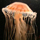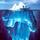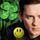## General Question# Complex-ish physics/maths question on the speed a car would need to take to go around a loop.

Asked by dotlin (419) August 6th, 2010

I basically want to know what formula they used in this video what steps did he take, so I could work out similar problems.
http://www.youtube.com/watch?v=wiZoVAZGgsw
I know basic projectile motion and masses on inclined planes, etc the basic stuff but I haven’t come across a question like this before.

Observing members: 0Composing members: 0## 2 AnswersThe acceleration of circular motion is ( v ^2 / r ) where v = speed and r = radius of track. Because the track is vertical, the acceleration at the top must exceed the acceleration due to gravity. Otherwise the car falls off the track. So the critical velocity is given by:

v^2 / r = g where g = gravitational acceleration. So v^2 = g * r, and v = sq.root (g * r). As long as v is greater than this value the loop-the-loop is safely executed.

The car is always falling due to gravity. The trick is to go fast enough so the track is pushing downward on the car harder than gravity is pulling downward. From the viewpoint of the driver, centrifugal force at the apex exceeds gravity.

gasman (11296)“Great Answer” (5) Flag as…I’m with @gasman on this. At the top, you want to have the centripetal acceleration exceed g,. 9.8m/sec^2 or 32.2 ft/sec^2. I figure the driver experiences 2 g max at the bottom and near 0g at the top. I don’t understand why they bothered to take him up in a plane with 6 g’s unless it was just for the show.

LuckyGuy (36618)“Great Answer” (0) Flag as…## Answer this questionor

#### Join

to answer.

This question is in the General Section. Responses must be helpful and on-topic.

Your answer will be saved while you login or join.
Have a question? Ask Fluther!
What do you know more about?
or
Knowledge Networking @ Fluther